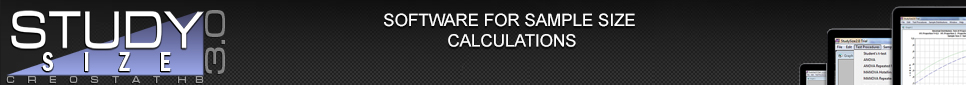### Tests and Other Procedures

 Parametric tests included in version 3.0. The sample size calculation can be performed for testing a one-sided (superiority, non-inferiority), two-sided (difference), or two one-sided (quivalence) hypothesis. For each test, different options are available for the parameters used in the calculations. Student's t-test Unpaired Paired ANOVA One-way Two-way Three-way Single Latin Square Replicated Latin Squares Replicated Latin Squares (block factor) Randomised Blocks, one factor Randomised Blocks, two factors ANOVA Repeated Measures One within factor One within, one between factor MANOVA Hotelling's T2 One groups Two groups MANOVA Repeated Measures One within factor One within, one between factor ANCOVA McNemar's test Paired Proportions test Stuart-Maxwell test Fisher's exact test Chi-square test 1 x C table, Goodness of Fit R x C table, Two Proportions R x C table, Homogeneity R x C table, Independence Mantel-Haenszel test Cochran-Armitage Trend test Exponential Survival (MLE) Hazard Difference test (Lachin-Foulkes) Hazard Ratio test (George-Desu) Hazard Ratio test (Rubenstein et.al.) Linear Regression Simple Multiple Logistic Regression Continuous covariate Binary covariate Poisson Regression Continuous covariate Binary covariate Pearson Correlation test Two-way Cross-Over Design Additive Model Multiplicative Model General Cross-Over Design Additive Model Multiplicative Model Goodness of Fit test Chi-square test Kolmogorov-Smirnov test
New in Version 3.0 .